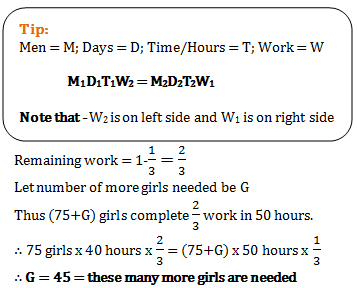# Aptitude Test for MBA students - Set 1

1)   The price of apple is first increased by 10% and then decreased by 10%. What is the change in the price of apple?

a. 1.11%
b. 3.5%
c. 5%
d. 1%
 Answer  Explanation ANSWER: 1% Explanation: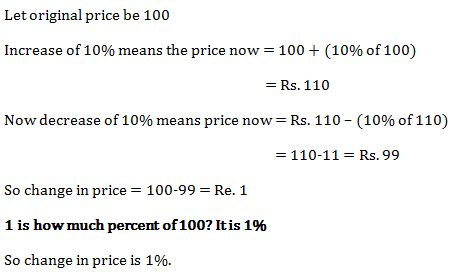2)   The population of a city is 50,000 at present. It increases at the rate of 10% per annum. What will be its population 3 yr from now?

a. 67,250
b. 65,550
c. 60,500
d. 66,550
 Answer  Explanation ANSWER: 66,550 Explanation: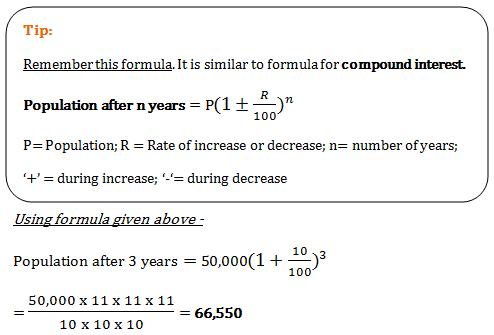3)   Income ratio of Ramesh and Suresh is 5:6. Their spending ratio is 7:9. Ramesh saves 4000 and Suresh saves 3000. Income and spending respectively of Ramesh and Suresh are

a. Ramesh - 25000, 21000; Suresh – 30000, 27000
b. Ramesh - 36000, 32000; Suresh – 30000, 27000
c. Ramesh - 30000, 27000; Suresh – 36000, 32000
d. None of the above
 Answer  Explanation ANSWER: Ramesh - 25000, 21000; Suresh – 30000, 27000 Explanation: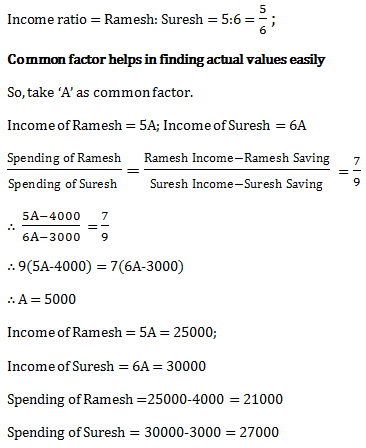4)   In 40 years an amount becomes 6 times the original amount. What is the rate of interest?

a. 12.5%
b. 24%
c. 6.67%
d. 34%
 Answer  Explanation ANSWER: 12.5% Explanation: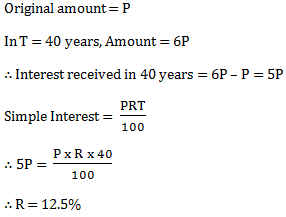5)   How much money invested at compound interest will yield Rs. 6350.40 at the end of 3 years? For the first year the rate of interest is 5%; for second year it increases to 12% and for third year it decreases to 8%.

a. Rs. 5800
b. Rs. 5500
c. Rs. 6000
d. Rs. 5000
 Answer  Explanation ANSWER: Rs. 5000 Explanation: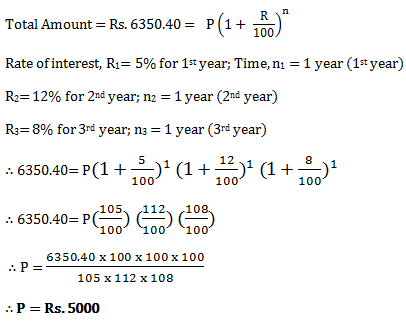6)   Amit gave his friend Rs. 11000. This was to be repaid in 2 yearly installments with a rate of interest of 20% compounded annually. How much would be the value of each installment?

a. Rs. 7200
b. Rs. 8200
c. Rs. 5500
d. Rs. 6400
 Answer  Explanation ANSWER: Rs. 7200 Explanation: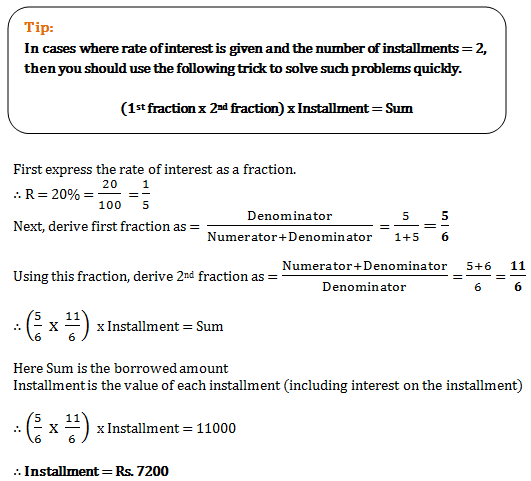7)   Surendra covers a distance by cycle at 18 km/h.  He returns to the starting point in a bus at a speed of 45 km/h. Find the average speed for the entire journey.

a. 27.39 km/hr
b. 25.71 km/hr
c. 27 km/hr
d. 31.5km/hr
 Answer  Explanation ANSWER: 25.71 km/hr Explanation: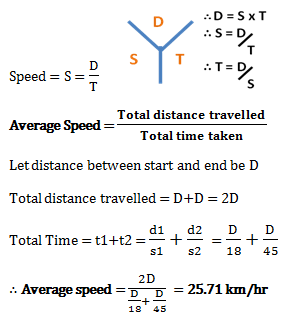8)   Ram and Shyam’s average age is 65 years. The average age of Ram, Shyam and John is 53 years. What is the age of John?

a. 29 years
b. 31 years
c. 59 years
d. 45 years
 Answer  Explanation ANSWER: 29 years Explanation: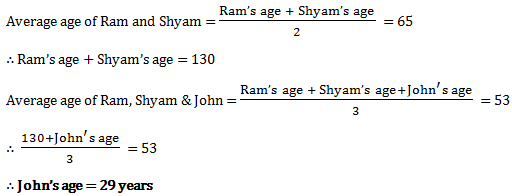9)   A can do a work in 3 days. B can do the same work in 6 days and C can do the same work in 7 days. If they work together, in how many days will they take to complete the work?

a. 11/10 Days
b. 15/16 Days
c. 14/9 Days
d. 16/7 Days
 Answer  Explanation ANSWER: 14/9 Days Explanation: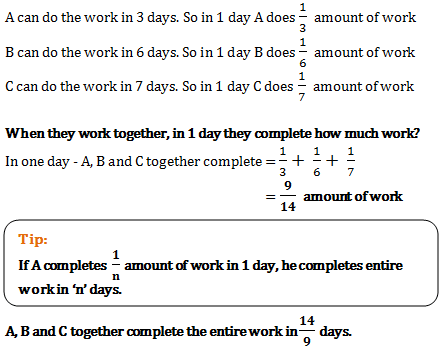10)   When P alone does a work, he takes 25 days more than the time taken by P and Q working together to complete the work. But Q alone takes 9 days more than the time taken by P and Q working together to complete the work. In what time, P and Q together finish this work?

a. 18 days
b. 20 days
c. 25 days
d. 15 days
 Answer  Explanation ANSWER: 15 days Explanation: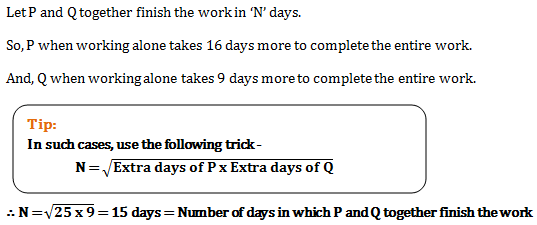11)   Ramesh can do a work in 5 days. Suresh can do the same work in 7 days. The total amount given for this work to them is Rs. 480. If both are working together, then what will be the share of Suresh?

a. Rs. 180
b. Rs. 300
c. Rs. 280
d. Rs. 200
 Answer  Explanation ANSWER: Rs. 200 Explanation: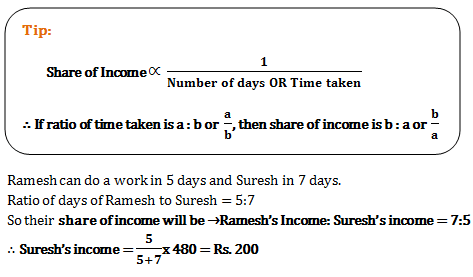12)   Parthiv was appointed for a 100 days job. The condition was that he will be paid Rs. 24 for every working day. But he will also be fined Rs.12 for every day he is absent. At the end he got Rs.420. For how many days, he was absent?

a. 45 Days
b. 55 Days
c. 75 Days
d. 64 Days
 Answer  Explanation ANSWER: 55 Days Explanation: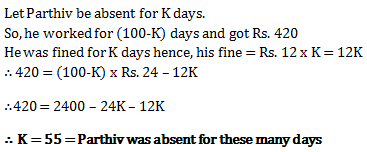13)   Pipe A can fill a tank 5 times faster than Pipe B and takes 32 minutes less than Pipe B to fill the tank. If both the pipes are opened together, then in how much time the tank would be full?

a. 32/3 minutes
b. 32/5 minutes
c. 20/3 minutes
d. 5/32 minutes
 Answer  Explanation ANSWER: 20/3 minutes Explanation: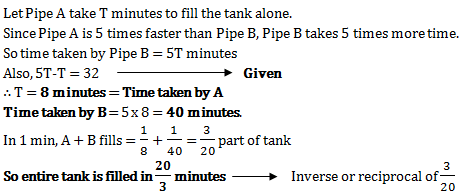14)   A train passes a stationary pole in 8 seconds. The train also passes a 200 m long bridge in 28 seconds. What is the length and the speed of the train?

a. Length = 200m; Speed = 36 m/s
b. Length = 160m; Speed = 20 m/s
c. Length = 80m; Speed = 10 m/s
d. Length = 100m; Speed = 20 m/s
 Answer  Explanation ANSWER: Length = 80m; Speed = 10 m/s Explanation: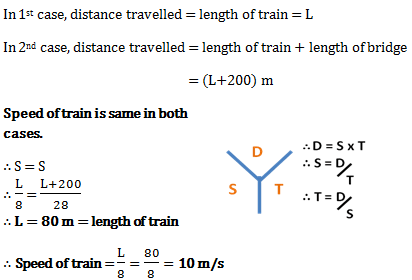15)   A train travels between P and Q in 3 hours. When the speed of train is increased by 6 km/hr, then it covers the same distance in 2 hours. What is original speed of train? Also find the distance between P and Q?

a. Distance = 48 km; Speed = 16 km/hr
b. Distance = 36 km; Speed = 12 km/hr
c. Distance = 51 km; Speed = 17 km/hr
d. Distance = 54 km; Speed = 18 km/hr
 Answer  Explanation ANSWER: Distance = 36 km; Speed = 12 km/hr Explanation: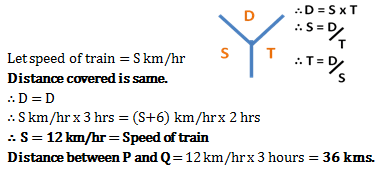16)   What is speed of Ram in still water and speed of river, if Ram covers 20 km in 1 hour swimming downstream and covers the same distance in 2 h swimming upstream?

a. River = 6 km/hr ; Ram = 14 km/hr
b. River = 8 km/hr ; Ram = 12 km/hr
c. River = 4 km/hr ; Ram = 16 km/hr
d. River = 5 km/hr ; Ram = 15 km/hr
 Answer  Explanation ANSWER: River = 5 km/hr ; Ram = 15 km/hr Explanation: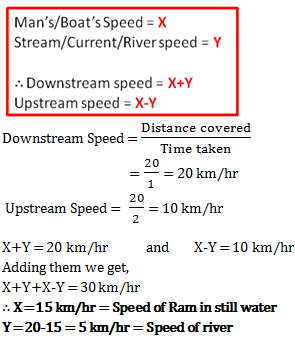17)   Of the 20 cycles sold by Ajay, average cost of 12 cycles is Rs. 18000. In total he earned Rs. 300000. What was the average cost of remaining cycles?

a. Rs. 10500
b. Rs. 7500
c. Rs. 9125
d. Rs. 9750
 Answer  Explanation ANSWER: Rs. 10500 Explanation: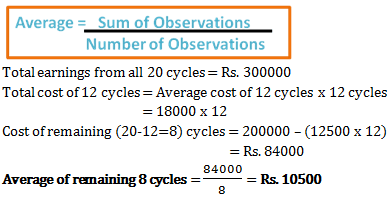18)   A batsman played 11 innings and has a certain average. This average increases by 2 runs when his 3 innings of 32 runs, 33 runs and 34 runs are replaced by 3 other innings. Find the average of these 3 new innings.

a. 40 runs
b. 35 (3/8) runs
c. 42 (3/8) runs
d. 40 (1/3) runs
 Answer  Explanation ANSWER: 40 (1/3) runs Explanation: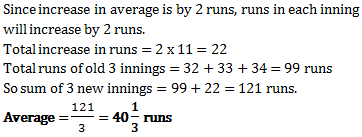19)   20 fishermen can catch 20 fish in 20 minutes. As per this, how many fish can 400 fishermen catch in 6 hours 40 minutes?

a. 8000
b. 4000
c. 20
d. 2000
 Answer  Explanation ANSWER: 8000 Explanation: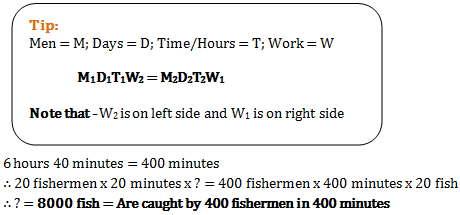20)   75 girls complete one-third decoration of a building in 40 hours. Now they have only 50 hours to complete the rest of the decoration. How many more girls are necessary to help them complete the task?

a. 45 girls
b. 150 girls
c. 100 girls
d. 55 girls
 Answer  Explanation ANSWER: 45 girls Explanation: